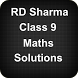# RD Sharma Class 9 Maths SolutionsFree App

Rated 0.00/5 (0) —  Free Android application by Apps4India

## About RD Sharma Class 9 Maths Solutions

RD Sharma Class 9 Maths Solutions app is specially designed for the CBSE class 9 students to help them prepare for their exams. It covers the latest CBSE Syllabus for Class 9th. This app also helps students throughout the year to complete their homework on time and double check the answers.

This app having Maths Class 9 solutions can be useful in two scenarios:
- Check the CBSE Maths Class 9 Solutions when you stuck solving the problems of the exercise
- To verify answers after you solve the exercise problems

This app contains answers of all the chapters included in the RD Sharma Class 9 Book:
Chapter 1 - Number System
Chapter 2 - Exponents of Real Numbers
Chapter 3 - Rationalisation
Chapter 4 - Algebraic Identities
Chapter 5 - Factorization of Algebraic Expressions
Chapter 6 - Factorization of Polynomials
Chapter 7 - Introduction to Euclid's Geometry
Chapter 8 - Lines and Angles
Chapter 9 - Triangle and its Angles
Chapter 10 - Congruent Triangles
Chapter 11 - Coordinate Geometry
Chapter 12 - Heron's Formula
Chapter 13 - Linear Equations in Two Variables
Chapter 15 - Areas of Parallelograms and Triangles
Chapter 16 - Circles
Chapter 17 - Construction
Chapter 18 - Surface Area and Volume of a Cuboid and Cube
Chapter 19 - Surface Area and Volume of A Right Circular Cylinder
Chapter 20 - Surface Area and Volume of A Right Circular Cone
Chapter 21 - Surface Area and Volume of a Sphere
Chapter 22 - Tabular Representation of Statistical Data
Chapter 23 - Graphical Representation of Statistical Data
Chapter 24 - Measures of Central Tendency
Chapter 25 - Probability

Features
- Expert Maths Solutions for all the exercises. It is your best study guide.
- The app is lightweight and doesn't occupy a lot of space on your phone.
- Use the RD Sharma Class 9 Maths solutions app without having an internet connection.

Free
downl.
Android
4.0+
For everyone
Android app

What's Changed
Initial release of the app

Oh snap! No comments are available for RD Sharma Class 9 Maths Solutions at the moment. Be the first to leave one!

RATING
0.05
0 users

5

4

3

2

1